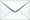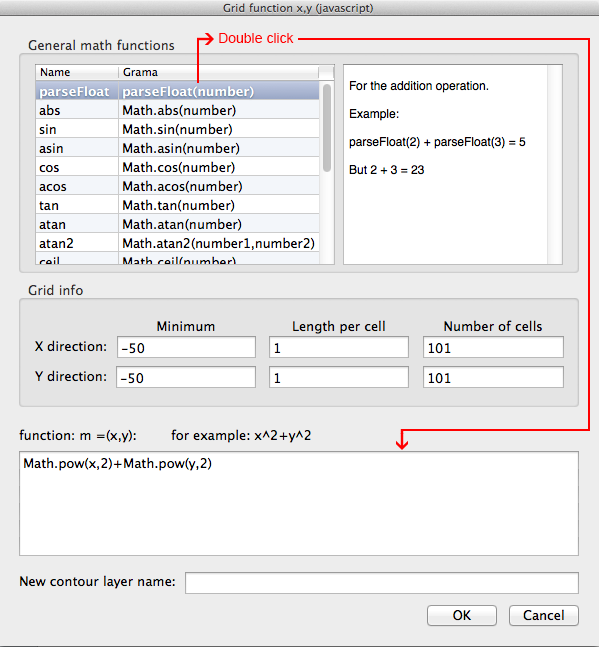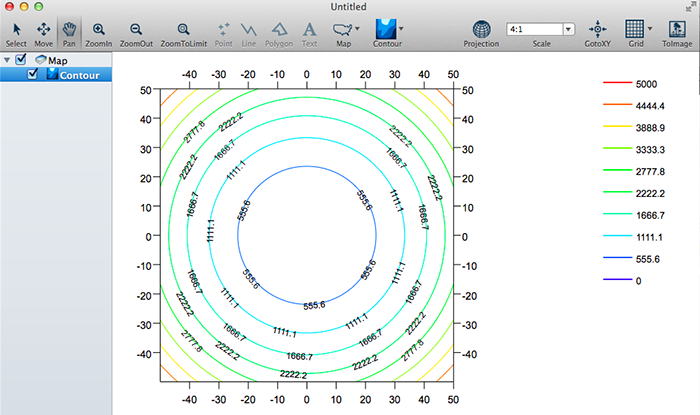Product Search

Dear customers:

take action as soon as possible.tyevolution@yahoo.com

EC: Contour__Grid function

Views:88times

Summarize:

Function command allows you to create a grid file from a user-defined two variable equation of the form M = f(X,Y). The density of the generated grid is a function of the Minimum, Number of cells, and Increment values for both X and Y. Function command can use any of the mathematical functions.

1. Function Dialog

Click [Contour][Grid function] to open the [Grid function x,y (javascript)] dialog.2. Enter a Function

Enter the function of interest into the a function of the form m = f(x,y) box. The written form of the function

considers General math functions. The function calculation is repeated for each M value to be written to the

grid file. The number of grid nodes in the output grid and, therefore, the number of calculations to be

performed, is based on the Minimum, Length per cell and Number of cells specified.

3. Minimum X and Y

In the Minimum X and Y boxes, specify the beginning values used in the specified function. The first grid node,

defined by these boxes,  is calculated based on these values. These values also specify the lowerX and Y

limits in the grid file.

4. Length per cell X and Y

In the Length per cell X and Y boxes, specify the amount to step in the X and Y directions for each grid line.

5. Number of cells X and Y

In the Number of cells X and Y boxes, specify number of steps in the X and Y directions for each grid line.

6. Output Grid

When done editing, click [OK]. Shown: